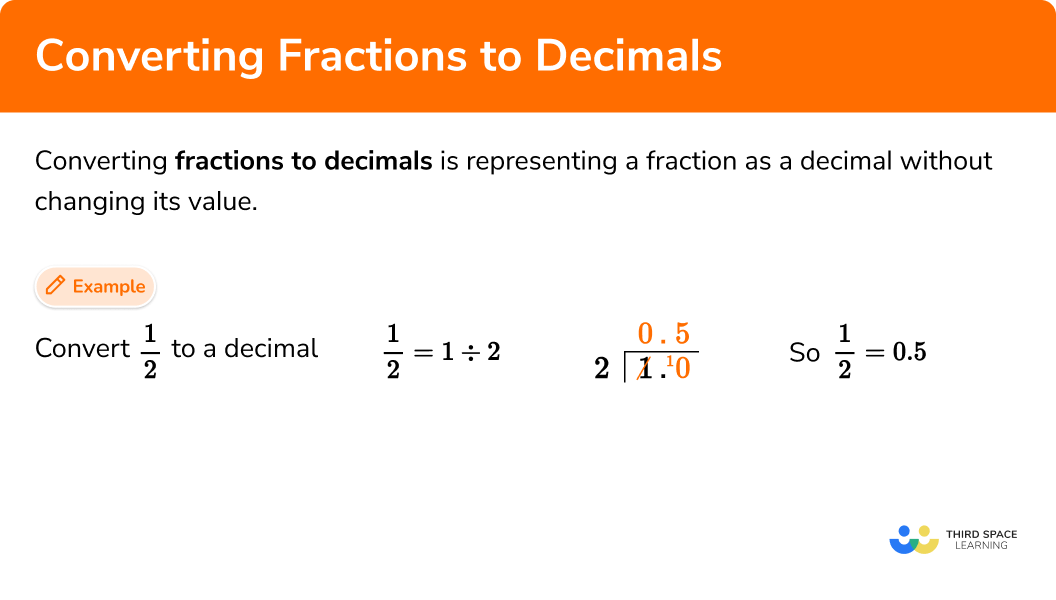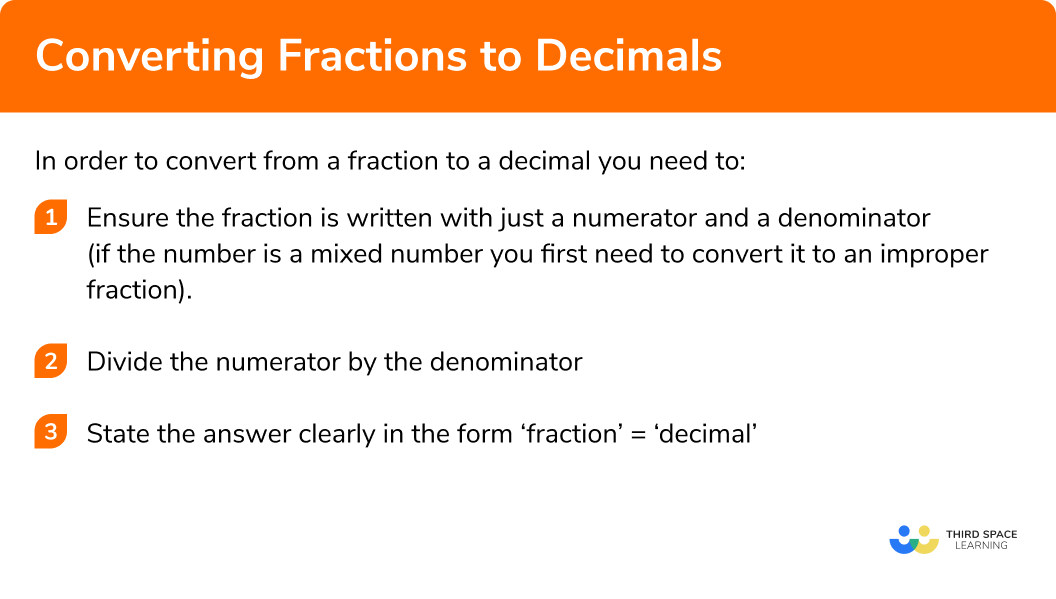GCSE Maths Number FDP Converting FDP

Fractions To Decimals

# Fractions To Decimals

Here we will learn about converting fractions to decimals.
There are also converting fractions to decimals worksheets based on Edexcel, AQA and OCR exam questions, along with further guidance on where to go next if you’re still stuck.

## What is converting fractions to decimals?

Converting fractions to decimals is representing a fraction as a decimal without changing its value.

E.g.

\begin{aligned} &\frac{1}{4}=0.25\\\\ &\frac{1}{8}=0.125\\\\ &\frac{2}{7}=0.2857142857…\\\\ &3 \frac{1}{5}=3.2 \end{aligned}

### What is converting fractions to decimals?## How to convert fractions to decimals

In order to convert from a fraction to a decimal you need to:

1. Ensure the fraction is written with just a numerator and a denominator.
(if the number is a mixed number you first need to convert it to an improper fraction).
2. Divide the numerator by the denominator.
3. State the answer clearly in the form ‘fraction’=’decimal’.

### Explain how to convert from a fraction to a decimal in 3 steps## Converting fractions to decimals examples

### Example 1: converting a simple fraction to a decimal

Convert \frac{1}{2} to a decimal.

1. If needed convert the mixed number to an improper fraction.

There is no need to convert as the fraction is already in the correct form.

2Divide the numerator by the denominator.

Using the ‘bus stop method’:

3State the answer clearly in the form ‘fraction’=’decimal’.

\frac{1}{2} = 0.5

### Example 2: Converting a fraction to a decimal

Convert \frac{3}{8} to a decimal

There is no need to convert as the fraction is already in the correct form.

3 \div 8

\frac{3}{8}=0.375

### Example 3: Converting an improper fraction to a decimal

Convert \frac{7}{5} to a decimal

There is no need to convert as the fraction is already in the correct form.

7 \div 5

\frac{7}{5}=1.4

### Example 4: Converting a mixed number to a decimal

Convert 3 \frac{1}{4} to a decimal

You need to convert this mixed number to an improper fraction.

3 \frac{1}{4}= \frac{13}{4}

Step by step guide: Improper fractions and mixed numbers (coming soon)

13 \div 4

3 \frac{1}{4}=3.25

### Example 5: Converting a fraction to a decimal involving a recurring decimal

Convert \frac{2}{9} to a decimal.

There is no need to convert as the fraction is already in the correct form.

2 \div 9

You will notice here that the 2 is repeated and will continue to be repeated. This is therefore a recurring decimal and can be shown in the form 0.\dot{2} .

\frac{2}{9}=0 . \dot{2}

### Example 6: Converting a mixed number to a decimal involving a recurring decimal

Convert 2\frac{1}{7} to a decimal.

You need to convert this mixed number to an improper fraction.

2\frac{1}{7}=\frac{15}{7}

Step by step guide: Improper fractions and mixed numbers (coming soon).

You will notice here that the 1 4 2 8 5 7 after the decimal place is repeated and will continue to be repeated. This is therefore a recurring decimal and can be shown as 2.\dot{1}4285\dot{7} .

2\frac{1}{7}=2 . \dot{1}4285\dot{7}

### Calculator skills

You can check your answer on a calculator by inputting the fraction into your calculator and then pressing the [S ⇔ D] button.

E.g.
Convert \frac{5}{4} to a decimal.

1. Press the fraction button on the left hand side of your calculator.

2. Input the numbers and press the = button.

3. Press the  [S ⇔ D] button to have it shown as a decimal.

Therefore \frac{5}{4}=1.25 .

### Common misconceptions

• Converting between a mixed number and an improper fraction

A common error is to incorrectly convert between numbers in different forms
E.g.
Mixed numbers and improper fractions.

• Written division

A common error is to incorrectly use a written method of division
E.g. the bus stop method or other methods of long division.

• Division

A common error is to divide the denominator by the numerator rather than dividing the numerator by the denominator.
E.g.
\frac{5}{7} means 5 \div 7 not 7 \div 5

Fractions to decimals is part of our series of lessons to support revision on comparing fractions, decimals and percentages. You may find it helpful to start with the main comparing fractions, decimals and percentages lesson for a summary of what to expect, or use the step by step guides below for further detail on individual topics. Other lessons in this series include:

### Practice converting fractions to decimals questions

1. Convert \frac{1}{4} to a decimal

1.40.2540.41 ÷ 4 gives the decimal 0.25

2. Convert \frac{7}{8} to a decimal

1.\dot{1}4285\dot{7}0.780.8757.87 ÷ 8  gives the correct decimal 0.875

3. Convert \frac{7}{4} to a decimal

1.751\frac{3}{4}7.40.\dot{7}5142\dot{8}7 ÷ 4  gives the correct decimal 1.75

4. Convert 7\frac{3}{5} to a decimal

4.2\frac{21}{5}7.357.6Converting to an improper fraction gives \frac{38}{5} , then you divide the numerator by the denominator.

5. Convert \frac{4}{9} to a decimal

0.40.440.4440. \dot{4}Calculating 4\div9 gives the recurring decimal 0.\dot{4} . The dot above the 4 means that the 4 is repeated infinitely.

6. Convert \frac{2}{11} to a decimal

0.180.1818180.\dot{1}\dot{8}5.5Calculating 2\div11 gives the recurring decimal 0.\dot{1}\dot{8} . The dot above the 1 and 8 represents the 1 and 8 being repeated infinitely

### Converting fractions to decimals GCSE questions

1.  a) Convert \frac{1}{2} to a decimal

b) Convert \frac{1}{4} to a decimal

c) Convert \frac{3}{5} to a decimal

(3 marks)

a) 0.5

(1)

b) 0.25

(1)

c) 0.6

(1)

2.  a) Convert 2\frac{1}{2} to a decimal

b) Convert \frac{8}{5} to a decimal

c) Convert \frac{1}{3} to a decimal

(3 marks)

a) 2.5

(1)

b) 1.6

(1)

c) 0. \dot{3}

Must be shown to be recurring

Accept 0.333333…

(1)

3.  a) Convert \frac{7}{10} to a decimal

b) Convert \frac{2}{25} to a decimal

c) Convert \frac{2}{9} to a decimal

(3 marks)

a) 0.7

(1)

b) 0.08

(1)

c) 0. \dot{2}

Must be shown to be recurring

Accept 0.222…

(1)

## Learning checklist

You have now learned how to:

• Convert a fraction to a decimal
• Convert a fraction to a recurring decimal

## Still stuck?

Prepare your KS4 students for maths GCSEs success with Third Space Learning. Weekly online one to one GCSE maths revision lessons delivered by expert maths tutors.

Find out more about our GCSE maths tuition programme.